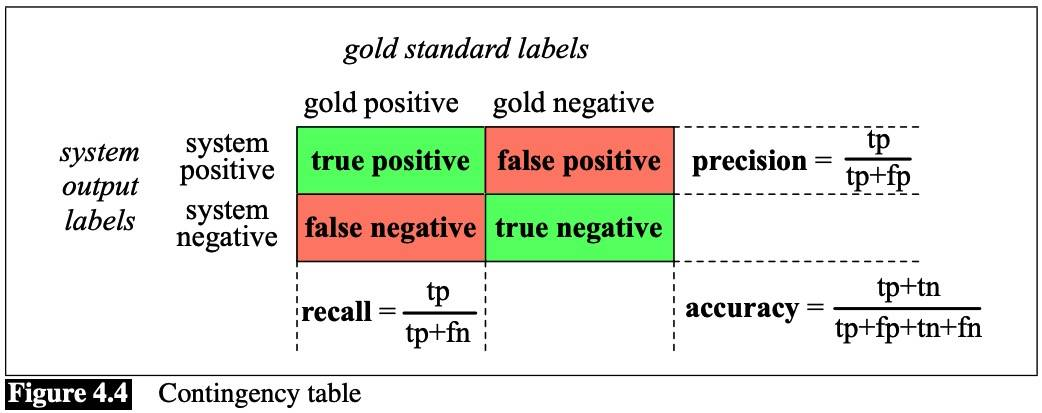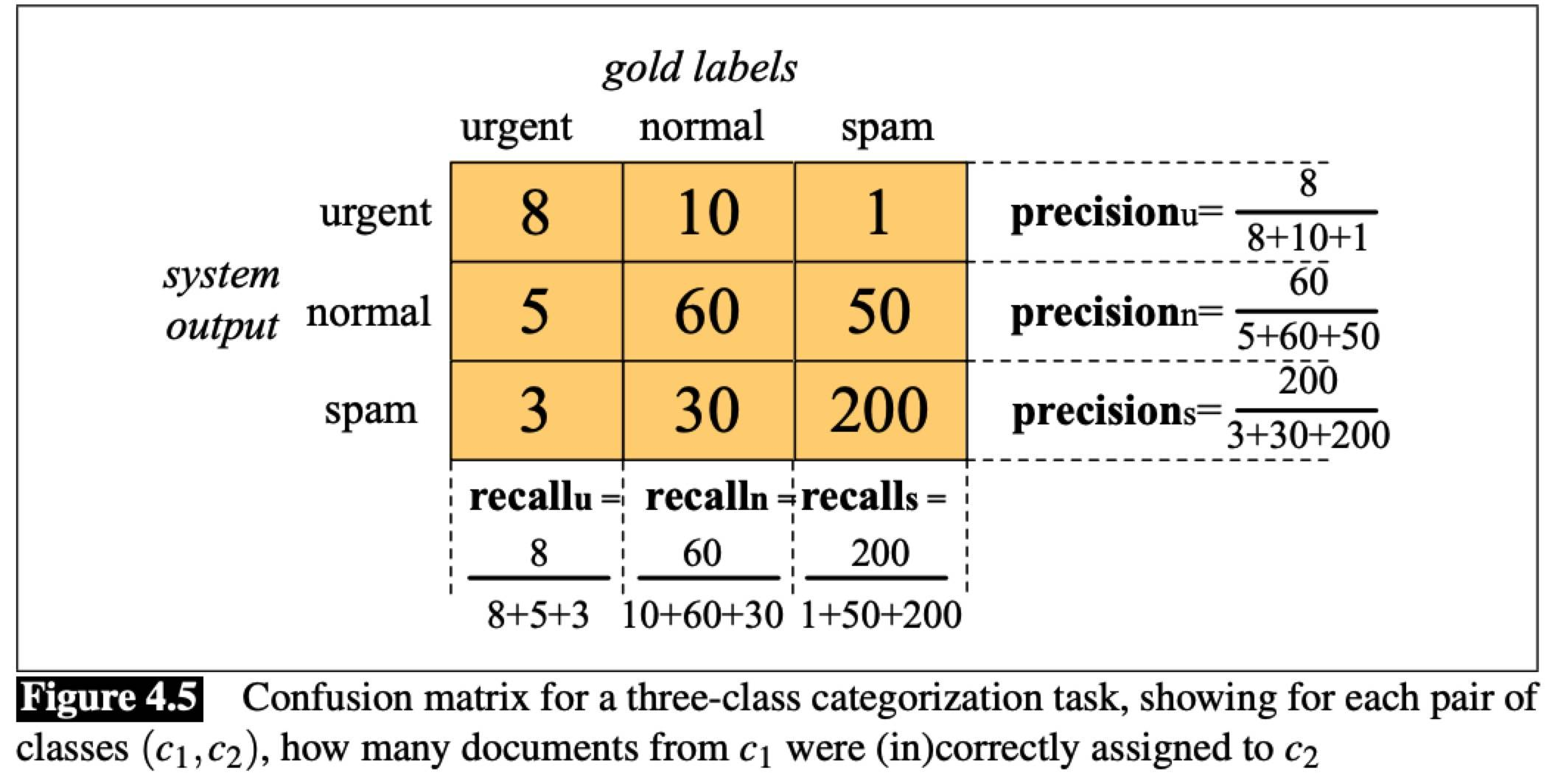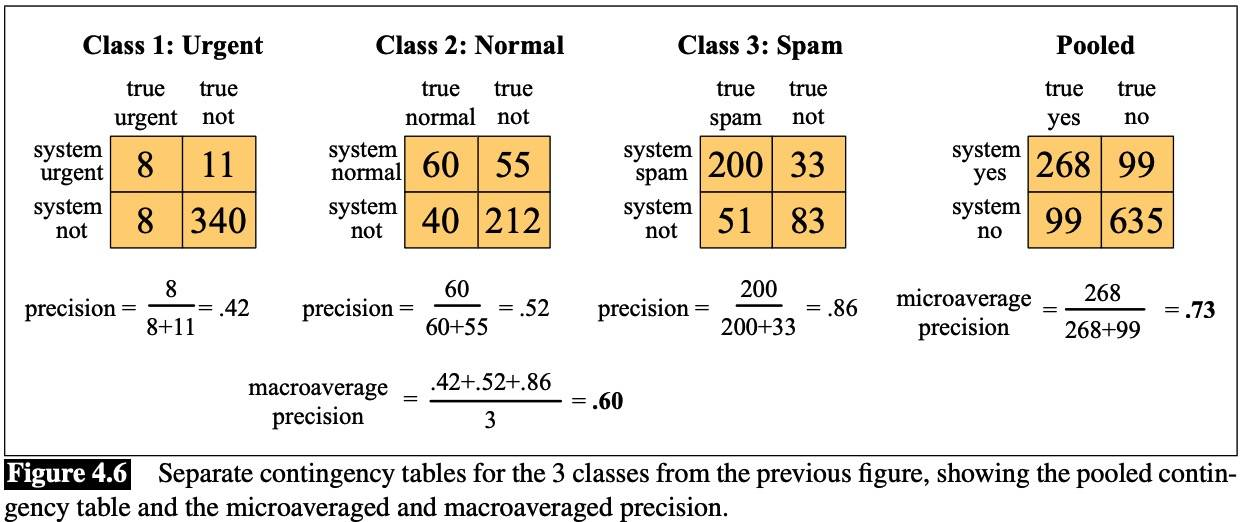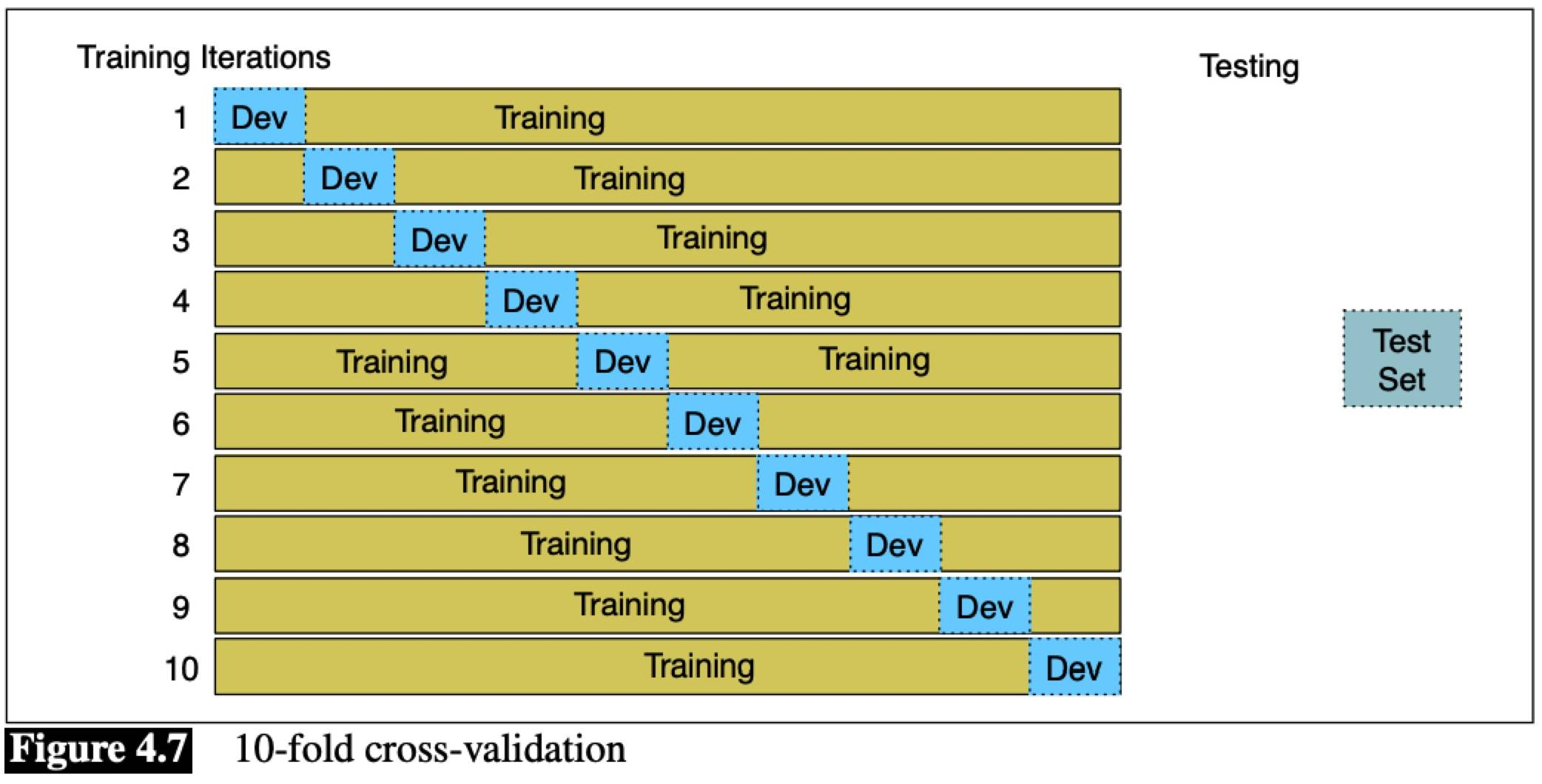# Naive Bayes and Sentiment Classification Note (SLP Ch04)

Text categorization, the task of assigning a label or category to an entire text or document.

• sentiment analysis
• spam detection
• subject category or topic label

Probabilistic classifier additionally will tell us the probability of the observation being in the class.

Generative classifiers like naive Bayes build a model of how a class could generate some input data.

Discriminative classifiers like logistic regression instead learn what features from the input are most useful to discriminate between the different possible classes.

## Naive Bayes Classifiers

Bayesian inference:

• given document d
• prior probability of the class P(c)
• likelihood of the document p(d|c)

• f1, f2, … as a set of features

P(f1,f2…|c) is hard to compute directly, estimate the probability of every possible combination of features (for example, every possible set of words and positions) would require huge numbers of parameters and impossibly large training sets.

Naive Bayes classifiers make two simplifying assumptions:

• position doesn’t matter
• P(fi|c) are independent given the class c: $P(f_1,f_2,…,f_n|c) = P(f_1|c)P(f_2|c)…P(f_n|c)$

To apply the naive Bayes classifier to text, we need to consider word position:

Calculations are done in log space to avoid underflow and increase speed:

## Training the Naive Bayes Classifer

If a word only occurs in one class, the probability for this feature in the other class will be zero, then the total class is zero, no matter the other evidence. The simplest solution is Add-one smoothing:

V consists of the union of all the word types in all classes, not just the words in one class c.

The solution for unknown words (in test data) is to ignore them, remove them from the test document and not include any probability for them at all.

In most text classification applications, however, using a stop word list doesn’t improve performance, and so it is more common to make use of the entire vocabulary and not use a stop word list.

The code is here: Naive-Bayes

## Optimizing for Sentiment Analysis

• In some tasks like sentiment classification, whether a word occurs or not seems to matter more than its frequency. Thus it often improve performance to clip the word counts in each document at 1. This variant is called binary multinomial naive Bayes or binary NB. DO NOT need to smooth. In Python, just use set(doc_i).

• Deal with negation. A simple baseline is to add a prefix NOT_ to every word after a token of logical negation (n’t, not, no, never) until the next punctuation mark. It works quite well in practice.

• When training data is insufficient, we can instead derive the positive and negative word features from sentiment lexicons.
• A common way to use lexicons is a naive Bayes classifier is to add a feature that is counted whenever a word from that lexicon occurs.
• If training data is sparse or not representative of the test set, using dense lexicon features (whether a word occurs in the lexicon) instead of sparse individual-word features may generalize better.

## Naive Bayes for other text classification tasks

• spam detection: sets of words or phrases as features
• language ID: byte n-grams.

## Naive Bayes as aLanguage Model

If we use all the words in the text, naive Bayes is similar to language model. Specifically, a naive Bayes model can be viewed as a set of class-specific unigram language models, in which the model for each class instantiates a unigram language model.

Since the likelihood features from the naive Bayes model assign a probability to each word P(w|c), the model also assigns a probability to each sentence: $P(s|c) = \prod_{i \in positions} P(w_i|c)$

## Evaluation: Precision, Recall, F-measure

Accuracy is not a good metric when the goal is to discover something that is rare, or at least not completely balanced in frequency, which is a very common situation in the world.

Precision and recall, emphasize true positives: finding the things that we are supposed to be looking for.

F-measure: $F_\beta = \frac {(\beta^2+1)PR}{\beta^2P + R}$

The β weights the importance of recall and precision, based on the needs of an application.

• β > 1 favor recall
• β < 1 favor precision
• β = 1 equally balanced, called F1

F-measure comes from a weighted harmonic mean of precision and recall.

### More than two classes

Two kinds of multi-class classification tasks:

• any-of
• multi-label

We combine the metric values in two ways:

• macroaveraging: compute the performance for each class, and then average over classes.
• microaveraging: collect the decisions for all classes into a single contingency table, and
then compute precision and recall from that table.## Test sets and Cross-validation

We use the development test set (devset) to tune some parameters.

If we could use all our data both for training and test, we do this by cross-validation:

• randomly choose a training and test set division of data, train and compute error rate
• repeat with a different randomly selected training and test set

Repeat times n, we get an average error rate, it is called n-fold cross-validation.

As all the data is used for test, we need the whole corpus to be blind, means we can’t examine any of the data to suggest possible features and see what’s going on. But looking at the corpus is often important.

So it is common to create a fixed training and test set, then do cross-validation inside the training set, but compute error rate the normal way in the test set.## Statistical Significance Testing

We have a test set x of n observations x = x1, x2, … xn on which A’s performance is better than B by δ(x). We need to reject the null hypothesis that A isn’t really better than B and this difference δ(x) occurred purely by chance.

In language processing we use non-parametric tests like the bootstrap test, or a similar test approximate randomization because most metrics are not normally distributed. Bootstrapping refers to repeatedly drawing large numbers of smaller samples with replacement (bootstrap samples) from an original larger sample.

The code is here: Statistical-Significance-Testing